# Tag: Riemann

Last time we revisited Robin’s theorem saying that 5040 being the largest counterexample to the bound
$\frac{\sigma(n)}{n~log(log(n))} < e^{\gamma} = 1.78107...$ is equivalent to the Riemann hypothesis.

There’s an industry of similar results using other arithmetic functions. Today, we’ll focus on Dedekind’s Psi function
$\Psi(n) = n \prod_{p | n}(1 + \frac{1}{p})$
where $p$ runs over the prime divisors of $n$. It is series A001615 in the online encyclopedia of integer sequences and it starts off with

1, 3, 4, 6, 6, 12, 8, 12, 12, 18, 12, 24, 14, 24, 24, 24, 18, 36, 20, 36, 32, 36, 24, 48, 30, 42, 36, 48, 30, 72, 32, 48, 48, 54, 48, …

and here’s a plot of its first 1000 values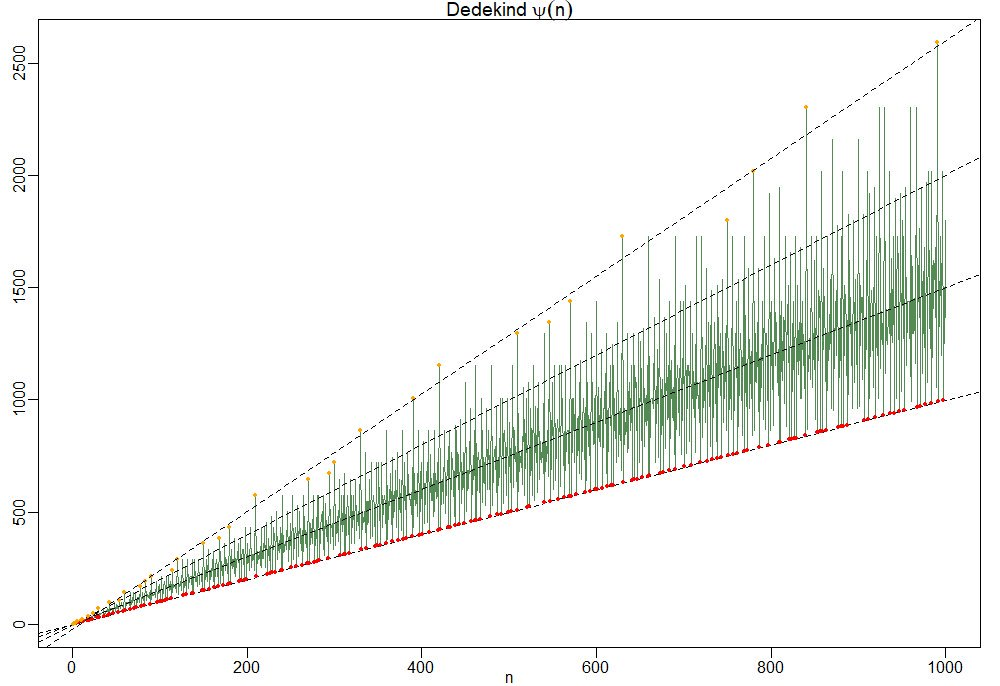To understand this behaviour it is best to focus on the ‘slopes’ $\frac{\Psi(n)}{n}=\prod_{p|n}(1+\frac{1}{p})$.

So, the red dots of minimal ‘slope’ $\approx 1$ correspond to the prime numbers, and the ‘outliers’ have a maximal number of distinct small prime divisors. Look at $210 = 2 \times 3 \times 5 \times 7$ and its multiples $420,630$ and $840$ in the picture.

For this reason the primorial numbers, which are the products of the fist $k$ prime numbers, play a special role. This is series A002110 starting off with

1, 2, 6, 30, 210, 2310, 30030, 510510, 9699690, 223092870,…

In Patrick Solé and Michel Planat Extreme values of the Dedekind $\Psi$ function, it is shown that the primorials play a similar role for Dedekind’s Psi as the superabundant numbers play for the sum-of-divisors function $\sigma(n)$.

That is, if $N_k$ is the $k$-th primorial, then for all $n < N_k$ we have that the 'slope' at $n$ is strictly below that of $N_k$ $\frac{\Psi(n)}{n} < \frac{\Psi(N_k)}{N_k}$ which follows immediately from the fact that any $n < N_k$ can have at most $k-1$ distinct prime factors and $p \mapsto 1 + \frac{1}{p}$ is a strictly decreasing function.

Another easy, but nice, observation is that for all $n$ we have the inequalities
$n^2 > \phi(n) \times \psi(n) > \frac{n^2}{\zeta(2)}$
where $\phi(n)$ is Euler’s totient function
$\phi(n) = n \prod_{p | n}(1 – \frac{1}{p})$
This follows as once from the definitions of $\phi(n)$ and $\Psi(n)$
$\phi(n) \times \Psi(n) = n^2 \prod_{p|n}(1 – \frac{1}{p^2}) < n^2 \prod_{p~\text{prime}} (1 - \frac{1}{p^2}) = \frac{n^2}{\zeta(2)}$ But now it starts getting interesting.

In the proof of his theorem, Guy Robin used a result of his Ph.D. advisor Jean-Louis Nicolasknown as Nicolas’ criterion for the Riemann hypothesis: RH is true if and only if for all $k$ we have the inequality for the $k$-th primorial number $N_k$
$\frac{N_k}{\phi(N_k)~log(log(N_k))} > e^{\gamma}$
From the above lower bound on $\phi(n) \times \Psi(n)$ we have for $n=N_k$ that
$\frac{\Psi(N_k)}{N_k} > \frac{N_k}{\phi(N_k) \zeta(2)}$
and combining this with Nicolas’ criterion we get
$\frac{\Psi(N_k)}{N_k~log(log(N_k))} > \frac{N_k}{\phi(N_k)~log(log(N_k)) \zeta(2)} > \frac{e^{\gamma}}{\zeta(2)} \approx 1.08…$
In fact, Patrick Solé and Michel Planat prove in their paper Extreme values of the Dedekind $\Psi$ function that RH is equivalent to the lower bound
$\frac{\Psi(N_k)}{N_k~log(log(N_k))} > \frac{e^{\gamma}}{\zeta(2)}$
holding for all $k \geq 3$.

Dedekind’s Psi function pops up in lots of interesting mathematics.

In the theory of modular forms, Dedekind himself used it to describe the index of the congruence subgroup $\Gamma_0(n)$ in the full modular group $\Gamma$.

In other words, it gives us the number of tiles needed in the Dedekind tessellation to describe the fundamental domain of the action of $\Gamma_0(n)$ on the upper half-plane by Moebius transformations.When $n=6$ we have $\Psi(6)=12$ and we can view its fundamental domain via these Sage commands:

 G=Gamma0(6) FareySymbol(G).fundamental_domain() 

giving us the 24 back or white tiles (note that these tiles are each fundamental domains of the extended modular group, so we have twice as many of them as for subgroups of the modular group)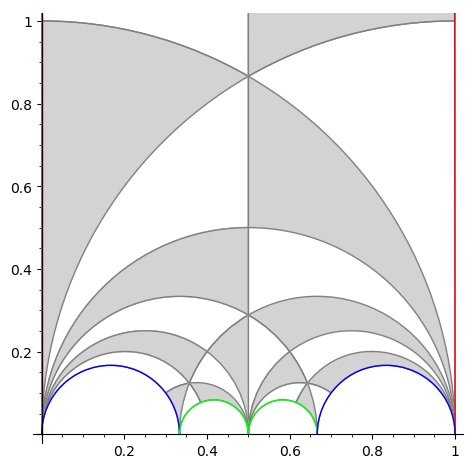But, there are plenty of other, seemingly unrelated, topics where $\Psi(n)$ appears. To name just a few:

• The number of points on the projective line $\mathbb{P}^1(\mathbb{Z}/n\mathbb{Z})$.
• The number of lattices at hyperdistance $n$ in Conway’s big picture.
• The number of admissible maximal commuting sets of operators in the Pauli group for the $n$ qudit.

and there are explicit natural one-to-one correspondences between all these manifestations of $\Psi(n)$, tbc.

“She simply walked into the pond in Kensington Gardens Sunday morning and drowned herself in three feet of water.”

This is the opening sentence of The Ishango Bone, a novel by Paul Hastings Wilson. It (re)tells the story of a young mathematician at Cambridge, Amiele, who (dis)proves the Riemann Hypothesis at the age of 26, is denied the Fields medal, and commits suicide.

In his review of the novel on MathFiction, Alex Kasman casts he story in the 1970ties, based on the admission of the first female students to Trinity.

More likely, the correct time frame is in the first decade of this century. On page 121 Amiele meets Alain Connes, said to be a “past winner of the Crafoord Prize”, which Alain obtained in 2001. In fact, noncommutative geometry and its interaction with quantum physics plays a crucial role in her ‘proof’.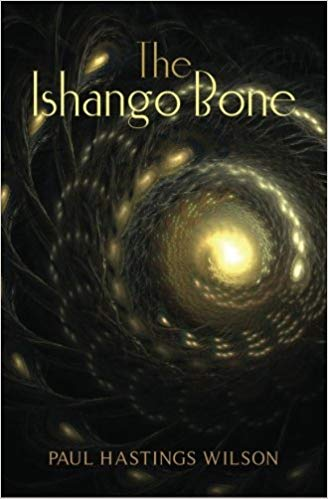The Ishango artefact only appears in the Coda to the book. There are a number of theories on the nature and grouping of the scorings on the bone. In one column some people recognise the numbers 11, 13, 17 and 19 (the primes between 10 and 20).

In the book, Amiele remarks that the total number of lines scored on the bone (168) “happened to be the exact total of all the primes between 1 and 1000” and “if she multiplied 60, the total number of lines in one side column, by 168, the grand total of lines, she’d get 10080,…,not such a far guess from 9592, the actual total of primes between 1 and 100000.” (page 139-140)

The bone is believed to be more than 20000 years old, prime numbers were probably not understood until about 500 BC…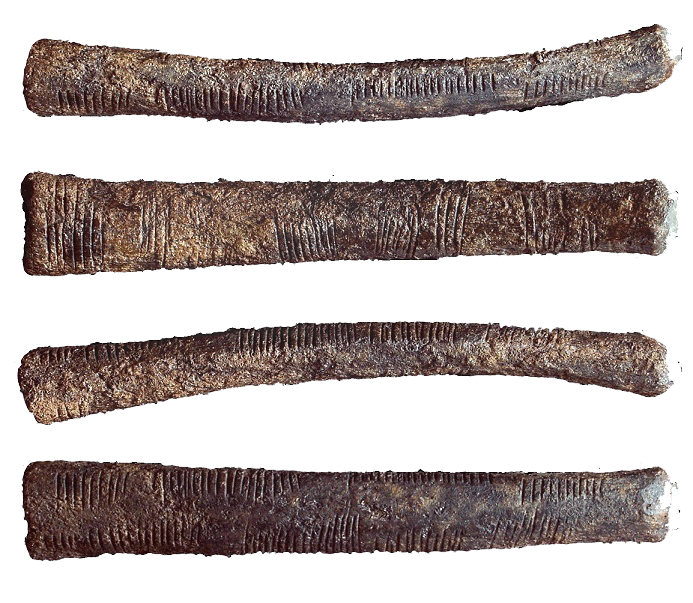More interesting than these speculations on the nature of the Ishango bone is the description of the tools Amiele thinks to need to tackle the Riemann Hypothesis:

“These included algebraic geometry (which combines commutative algebra with the language and problems of geometry); noncommutative geometry (concerned with the geometric approach to associative algebras, in which multiplication is not commutative, that is, for which $x$ times $y$ does not always equal $y$ times $x$); quantum field theory on noncommutative spacetime, and mathematical aspects of quantum models of consciousness, to name a few.” (page 115)

The breakthrough came two years later when Amiele was giving a lecture on Grothendieck’s dessins d’enfant.

“Dessin d’enfant, or ‘child’s drawing’, which Amiele had discovered in Grothendieck’s work, is a type of graph drawing that seemed technically simple, but had a very strong impression on her, partly due to the familiar nature of the objects considered. (…) Amiele found subtle arithmetic invariants associated with these dessins, which were completely transformed, again, as soon as another stroke was added.” (page 116)

Amiele’s ‘disproof’ of RH is outlined on pages 122-124 of “The Ishango Bone” and is a mixture of recognisable concepts and ill-defined terms.

“Her final result proved that Riemann’s Hypothesis was false, a zero must fall to the east of Riemann’s critical line whenever the zeta function of point $q$ with momentum $p$ approached the aelotropic state-vector (this is a simplification, of course).” (page 123)

More details are given in a footnote:

“(…) a zero must fall to the east of Riemann’s critical line whenever:

$\zeta(q_p) = \frac{( | \uparrow \rangle + \Psi) + \frac{1}{2}(1+cos(\Theta))\frac{\hbar}{\pi}}{\int(\Delta_p)}$

(…) The intrepid are invited to try the equation for themselves.” (page 124)

Wilson’s “The Ishango Bone” was published in 2012. A fair number of topics covered (the Ishango bone, dessin d’enfant, Riemann hypothesis, quantum theory) also play a prominent role in the 2015 paper/story by Michel Planat “A moonshine dialogue in mathematical physics”, but this time with additional story-line: monstrous moonshine

Such a paper surely deserves a separate post.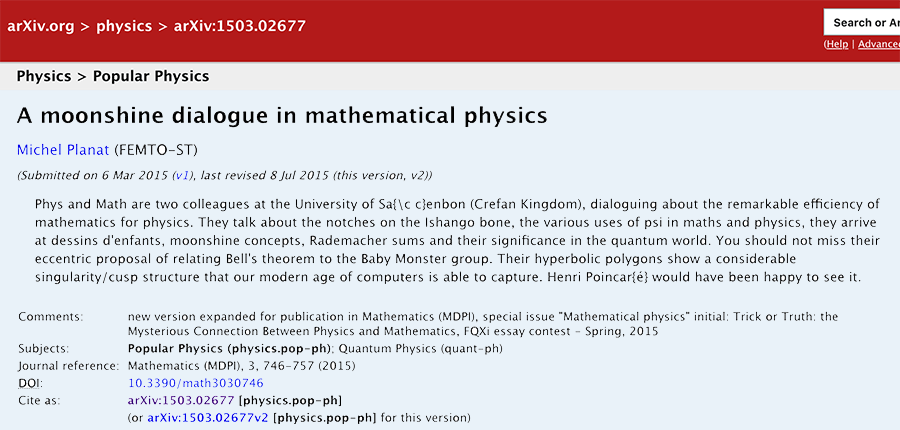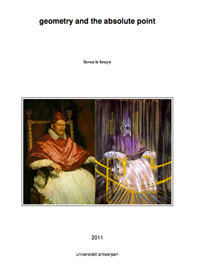In preparing for next year’s ‘seminar noncommutative geometry’ I’ve converted about 30 posts to LaTeX, centering loosely around the topics students have asked me to cover : noncommutative geometry, the absolute point (aka the field with one element), and their relation to the Riemann hypothesis.

The idea being to edit these posts thoroughly, add much more detail (and proofs) and also add some extra sections on Borger’s work and Witt rings (and possibly other stuff).

For those of you who prefer to (re)read these posts on paper or on a tablet rather than perusing this blog, you can now download the very first version (minimally edited) of the eBook ‘geometry and the absolute point’. All comments and suggestions are, of course, very welcome. I hope to post a more definite version by mid-september.

I’ve used the thesis-documentclass to keep the same look-and-feel of my other course-notes, but I would appreciate advice about turning LaTeX-files into ‘proper’ eBooks. I am aware of the fact that the memoir-class has an ebook option, and that one can use the geometry-package to control paper-sizes and margins.

Soon, I will be releasing a LaTeX-ed ‘eBook’ containing the Bourbaki-related posts. Later I might also try it on the games- and groups-related posts…

Previously, we have recalled comparisons between approaches to define a geometry over the absolute point and art-historical movements, first those due to Yuri I. Manin, subsequently some extra ones due to Javier Lopez Pena and Oliver Lorscheid.

In these comparisons, the art trend appears to have been chosen more to illustrate a key feature of the approach or an appreciation of its importance, rather than giving a visual illustration of the varieties over $\mathbb{F}_1$ the approach proposes.

Some time ago, we’ve had a couple of posts trying to depict noncommutative varieties, first the illustrations used by Shahn Majid and Matilde Marcolli, and next my own mental picture of it.

In this post, we’ll try to do something similar for affine varieties over the absolute point. To simplify things drastically, I’ll divide the islands in the Lopez Pena-Lorscheid map of $\mathbb{F}_1$ land in two subsets : the former approaches (all but the $\Lambda$-schemes) and the current approach (the $\Lambda$-scheme approach due to James Borger).

The former approaches : Francis Bacon “The Pope” (1953)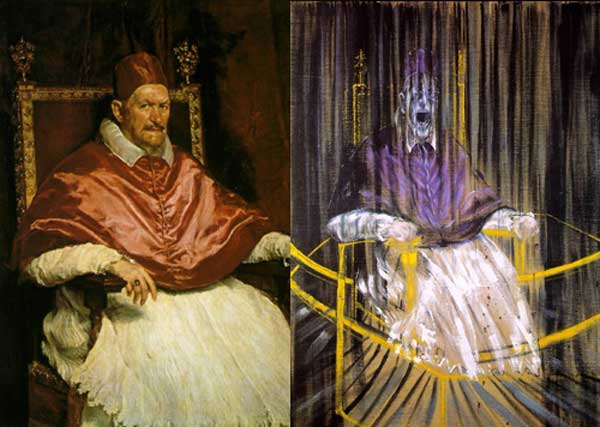The general consensus here was that in going from $\mathbb{Z}$ to $\mathbb{F}_1$ one looses the additive structure and retains only the multiplicative one. Hence, ‘commutative algebras’ over $\mathbb{F}_1$ are (commutative) monoids, and mimicking Grothendieck’s functor of points approach to algebraic geometry, a scheme over $\mathbb{F}_1$ would then correspond to a functor

$h_Z~:~\mathbf{monoids} \longrightarrow \mathbf{sets}$

Such functors are described largely by combinatorial data (see for example the recent blueprint-paper by Oliver Lorscheid), and, if the story would stop here, any Rothko painting could be used as illustration.

Most of the former approaches add something though (buzzwords include ‘Arakelov’, ‘completion at $\infty$’, ‘real place’ etc.) in order to connect the virtual geometric object over $\mathbb{F}_1$ with existing real, complex or integral schemes. For example, one can make the virtual object visible via an evaluation map $h_Z \rightarrow h_X$ which is a natural transformation, where $X$ is a complex variety with its usual functor of points $h_X$ and to connect both we associate to a monoid $M$ its complex monoid-algebra $\mathbb{C} M$. An integral scheme $Y$ can then be said to be ‘defined over $\mathbb{F}_1$’, if $h_Z$ becomes a subfunctor of its usual functor of points $h_Y$ (again, assigning to a monoid its integral monoid algebra $\mathbb{Z} M$) and $Y$ is the ‘best’ integral scheme approximation of the complex evaluation map.

To illustrate this, consider the painting Study after Velázquez’s Portrait of Pope Innocent X by Francis Bacon (right-hand painting above) which is a distorded version of the left-hand painting Portrait of Innocent X by Diego Velázquez.

Here, Velázquez’ painting plays the role of the complex variety which makes the combinatorial gadget $h_Z$ visible, and, Bacon’s painting depicts the integral scheme, build up from this combinatorial data, which approximates the evaluation map best.

All of the former approaches more or less give the same very small list of integral schemes defined over $\mathbb{F}_1$, none of them motivically interesting.

The current approach : Jackson Pollock “No. 8” (1949)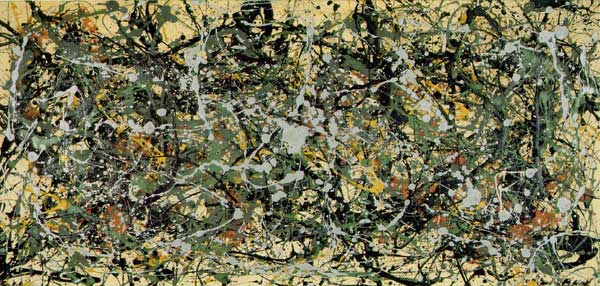An entirely different approach was proposed by James Borger in $\Lambda$-rings and the field with one element. He proposes another definition for commutative $\mathbb{F}_1$-algebras, namely $\lambda$-rings (in the sense of Grothendieck’s Riemann-Roch) and he argues that the $\lambda$-ring structure (which amounts in the sensible cases to a family of endomorphisms of the integral ring lifting the Frobenius morphisms) can be viewed as descent data from $\mathbb{Z}$ to $\mathbb{F}_1$.

The list of integral schemes of finite type with a $\lambda$-structure coincides roughly with the list of integral schemes defined over $\mathbb{F}_1$ in the other approaches, but Borger’s theory really shines in that it proposes long sought for mystery-objects such as $\mathbf{spec}(\mathbb{Z}) \times_{\mathbf{spec}(\mathbb{F}_1)} \mathbf{spec}(\mathbb{Z})$. If one accepts Borger’s premise, then this object should be the geometric object corresponding to the Witt-ring $W(\mathbb{Z})$. Recall that the role of Witt-rings in $\mathbb{F}_1$-geometry was anticipated by Manin in Cyclotomy and analytic geometry over $\mathbb{F}_1$.

But, Witt-rings and their associated Witt-spaces are huge objects, so one needs to extend arithmetic geometry drastically to include such ‘integral schemes of infinite type’. Borger has made a couple of steps in this direction in The basic geometry of Witt vectors, II: Spaces.

To depict these new infinite dimensional geometric objects I’ve chosen for Jackson Pollock‘s painting No. 8. It is no coincidence that Pollock-paintings also appeared in the depiction of noncommutative spaces. In fact, Matilde Marcolli has made the connection between $\lambda$-rings and noncommutative geometry in Cyclotomy and endomotives by showing that the Bost-Connes endomotives are universal for $\lambda$-rings.

Sunday january 2nd around 18hr NeB-stats went crazy.

Referrals clarified that the post ‘What is the knot associated to a prime?’ was picked up at Reddit/math and remained nr.1 for about a day.

Now, the dust has settled, so let’s learn from the experience.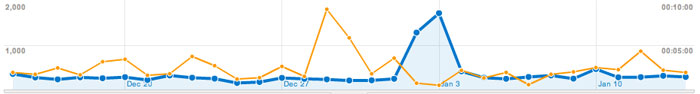A Reddit-mention is to a blog what doping is to a sporter.

You get an immediate boost in the most competitive of all blog-stats, the number of unique vistors (blue graph), but is doesn’t result in a long-term effect, and, it may even be harmful to more essential blog-stats, such as the average time visitors spend on your site (yellow graph).

For NeB the unique vistors/day fluctuate normally around 300, but peaked to 1295 and 1733 on the ‘Reddit-days’. In contrast, the avg. time on site is normally around 3 minutes, but dropped the same days to 44 and 30 seconds!

Whereas some of the Reddits spend enough time to read the post and comment on it, the vast majority zap from one link to the next. Having monitored the Reddit/math page for two weeks, I’m convinced that post only made it because it was visually pretty good. The average Reddit/math-er is a viewer more than a reader…

So, should I go for shorter, snappier, more visual posts?

Let’s compare Reddits to those coming from the three sites giving NeB most referrals : Google search, MathOverflow and Wikipedia.This is the traffic coming from Reddit/math, as always the blue graph are the unique visitors, the yellow graph their average time on site, blue-scales to the left, yellow-scales to the right.

Here’s the same graph for Google search. The unique visitors/day fluctuate around 50 and their average time on site about 2 minutes.The math-related search terms most used were this month : ‘functor of point approach’, ‘profinite integers’ and ‘bost-connes sytem’.

More rewarding to me are referrals from MathOverflow.The number of visitors depends on whether the MathO-questions made it to the front-page (for example, the 80 visits on december 15, came from the What are dessins d’enfants?-topic getting an extra comment that very day, and having two references to NeB-posts : The best rejected proposal ever and Klein’s dessins d’enfant and the buckyball), but even older MathO-topics give a few referrals a day, and these people sure take their time reading the posts (+ 5 minutes).

Other MathO-topics giving referrals this month were Most intricate and most beautiful structures in mathematics (linking to Looking for F-un), What should be learned in a first serious schemes course? (linking to Mumford’s treasure map (btw. one of the most visited NeB-posts ever)), How much of scheme theory can you visualize? (linking again to Mumford’s treasure map) and Approaches to Riemann hypothesis using methods outside number theory (linking to the Bost-Connes series).

Finally, there’s Wikipediagiving 5 to 10 referrals a day, with a pretty good time-on-site average (around 4 minutes, peaking to 12 minutes). It is rewarding to see NeB-posts referred to in as diverse Wikipedia-topics as ‘Fifteen puzzle’, ‘Field with one element’, ‘Evariste Galois’, ‘ADE classification’, ‘Monster group’, ‘Arithmetic topology’, ‘Dessin d’enfant’, ‘Groupoid’, ‘Belyi’s theorem’, ‘Modular group’, ‘Cubic surface’, ‘Esquisse d’un programme’, ‘N-puzzle’, ‘Shabat polynomial’ and ‘Mathieu group’.

What lesson should be learned from all this data? Should I go for shorter, snappier and more visual posts, or should I focus on the small group of visitors taking their time reading through a longer post, and don’t care about the appallingly high bounce rate the others cause?

Exactly 7 years ago I wrote my first post. This blog wasn’t called NeB yet and it used pMachine, a then free blogging tool (later transformed into expression engine), rather than WordPress.

Over the years NeB survived three hardware-upgrades of ‘the Matrix’ (the webserver hosting it), more themes than I care to remember, and a couple of dramatic closure announcements…

But then we’re still here, soldiering on, still uncertain whether there’s a point to it, but grateful for tiny tokens of appreciation.

Such as this morning’s story: Chandan deemed it necessary to correct two spelling mistakes in a 2 year old Fun-math post on Weil and the Riemann hypothesis (also reposted on Neb here). Often there’s a story behind such sudden comments, and a quick check of MathOverflow revealed this answer and the comments following it.

I thank Ed Dean for linking to the Fun-post, Chandan for correcting the misspellings and Georges for the kind words. I agree with Georges that a cut&copy of a blogpost-quoted text does not require a link to that post (though it is always much appreciated). It is rewarding to see such old posts getting a second chance…Above the Google Analytics graph of the visitors coming here via a mobile device (at most 5 on a good day…). Anticipating much more iPads around after tonights presents-session I’ve made NeB more accessible for iPods, iPhones, iPads and other mobile devices.

The first time you get here via your Mac-device of choice you’ll be given the option of saving NeB as an App. It has its own icon (lowest row middle, also the favicon of NeB) and flashy start-up screen.Of course, the whole point trying to make NeB more readable for Mobile users you get an overview of the latest posts together with links to categories and tags and the number of comments. Sliding through you can read the post, optimized for the device.I do hope you will use the two buttons at the end of each post, the first to share or save it and the second to leave a comment.

I wish you all a lot of mathematical (and other) fun in 2011 :: lieven.

This is a belated response to a Math-Overflow exchange between Thomas Riepe and Chandan Singh Dalawat asking for a possible connection between Connes’ noncommutative geometry approach to the Riemann hypothesis and the Langlands program.

Here’s the punchline : a large chunk of the Connes-Marcolli book Noncommutative Geometry, Quantum Fields and Motives can be read as an exploration of the noncommutative boundary to the Langlands program (at least for $GL_1$ and $GL_2$ over the rationals $\mathbb{Q}$).

Recall that Langlands for $GL_1$ over the rationals is the correspondence, given by the Artin reciprocity law, between on the one hand the abelianized absolute Galois group

$Gal(\overline{\mathbb{Q}}/\mathbb{Q})^{ab} = Gal(\mathbb{Q}(\mu_{\infty})/\mathbb{Q}) \simeq \hat{\mathbb{Z}}^*$

and on the other hand the connected components of the idele classes

$\mathbb{A}^{\ast}_{\mathbb{Q}}/\mathbb{Q}^{\ast} = \mathbb{R}^{\ast}_{+} \times \hat{\mathbb{Z}}^{\ast}$

The locally compact Abelian group of idele classes can be viewed as the nice locus of the horrible quotient space of adele classes $\mathbb{A}_{\mathbb{Q}}/\mathbb{Q}^{\ast}$. There is a well-defined map

$\mathbb{A}_{\mathbb{Q}}’/\mathbb{Q}^{\ast} \rightarrow \mathbb{R}_{+} \qquad (x_{\infty},x_2,x_3,\ldots) \mapsto | x_{\infty} | \prod | x_p |_p$

from the subset $\mathbb{A}_{\mathbb{Q}}’$ consisting of adeles of which almost all terms belong to $\mathbb{Z}_p^{\ast}$. The inverse image of this map over $\mathbb{R}_+^{\ast}$ are precisely the idele classes $\mathbb{A}^{\ast}_{\mathbb{Q}}/\mathbb{Q}^{\ast}$. In this way one can view the adele classes as a closure, or ‘compactification’, of the idele classes.

This is somewhat reminiscent of extending the nice action of the modular group on the upper-half plane to its badly behaved action on the boundary as in the Manin-Marcolli cave post.

The topological properties of the fiber over zero, and indeed of the total space of adele classes, are horrible in the sense that the discrete group $\mathbb{Q}^*$ acts ergodically on it, due to the irrationality of $log(p_1)/log(p_2)$ for primes $p_i$. All this is explained well (in the semi-local case, that is using $\mathbb{A}_Q’$ above) in the Connes-Marcolli book (section 2.7).

In much the same spirit as non-free actions of reductive groups on algebraic varieties are best handled using stacks, such ergodic actions are best handled by the tools of noncommutative geometry. That is, one tries to get at the geometry of $\mathbb{A}_{\mathbb{Q}}/\mathbb{Q}^{\ast}$ by studying an associated non-commutative algebra, the skew-ring extension of the group-ring of the adeles by the action of $\mathbb{Q}^*$ on it. This algebra is known to be Morita equivalent to the Bost-Connes algebra which is the algebra featuring in Connes’ approach to the Riemann hypothesis.

It shouldn’t thus come as a major surprise that one is able to recover the other side of the Langlands correspondence, that is the Galois group $Gal(\mathbb{Q}(\mu_{\infty})/\mathbb{Q})$, from the Bost-Connes algebra as the symmetries of certain states.

In a similar vein one can read the Connes-Marcolli $GL_2$-system (section 3.7 of their book) as an exploration of the noncommutative closure of the Langlands-space $GL_2(\mathbb{A}_{\mathbb{Q}})/GL_2(\mathbb{Q})$.

At the moment I’m running a master-seminar noncommutative geometry trying to explain this connection in detail. But, we’re still in the early phases, struggling with the topology of ideles and adeles, reciprocity laws, L-functions and the lot. Still, if someone is interested I might attempt to post some lecture notes here.

Next time you visit your math-library, please have a look whether these books are still on the shelves : Michiel Hazewinkel‘s Formal groups and applications, William Fulton’s and Serge Lange’s Riemann-Roch algebra and Donald Knutson’s lambda-rings and the representation theory of the symmetric group.

I wouldn’t be surprised if one or more of these books are borrowed out, probably all of them to the same person. I’m afraid I’m that person in Antwerp…

Lately, there’s been a renewed interest in $\lambda$-rings and the endo-functor W assigning to a commutative algebra its ring of big Witt vectors, following Borger’s new proposal for a geometry over the absolute point.However, as Hendrik Lenstra writes in his 2002 course-notes on the subject Construction of the ring of Witt vectors : “The literature on the functor W is in a somewhat unsatisfactory state: nobody seems to have any interest in Witt vectors beyond applying them for a purpose, and they are often treated in appendices to papers devoting to something else; also, the construction usually depends on a set of implicit or unintelligible formulae. Apparently, anybody who wishes to understand Witt vectors needs to construct them personally. That is what is now happening to myself.”

Before doing a series on Borger’s paper, we’d better run through Lenstra’s elegant construction in a couple of posts. Let A be a commutative ring and consider the multiplicative group of all ‘one-power series’ over it $\Lambda(A)=1+t A[[t]]$. Our aim is to define a commutative ring structure on $\Lambda(A)$ taking as its ADDITION the MULTIPLICATION of power series.

That is, if $u(t),v(t) \in \Lambda(A)$, then we define our addition $u(t) \oplus v(t) = u(t) \times v(t)$. This may be slightly confusing as the ZERO-element in $\Lambda(A),\oplus$ will then turn be the constant power series 1…

We are now going to define a multiplication $\otimes$ on $\Lambda(A)$ which is distributively with respect to $\oplus$ and turns $\Lambda(A)$ into a commutative ring with ONE-element the series $~(1-t)^{-1}=1+t+t^2+t^3+\ldots$.

We will do this inductively, so consider $\Lambda_n(A)$ the (classes of) one-power series truncated at term n, that is, the kernel of the natural augmentation map between the multiplicative group-units $~A[t]/(t^{n+1})^* \rightarrow A^*$.
Again, taking multiplication in $A[t]/(t^{n+1})$ as a new addition rule $\oplus$, we see that $~(\Lambda_n(A),\oplus)$ is an Abelian group, whence a $\mathbb{Z}$-module.

For all elements $a \in A$ we have a scaling operator $\phi_a$ (sending $t \rightarrow at$) which is an A-ring endomorphism of $A[t]/(t^{n+1})$, in particular multiplicative wrt. $\times$. But then, $\phi_a$ is an additive endomorphism of $~(\Lambda_n(A),\oplus)$, so is an element of the endomorphism-RING $End_{\mathbb{Z}}(\Lambda_n(A))$. Because composition (being the multiplication in this endomorphism ring) of scaling operators is clearly commutative ($\phi_a \circ \phi_b = \phi_{ab}$) we can define a commutative RING $E$ being the subring of $End_{\mathbb{Z}}(\Lambda_n(A))$ generated by the operators $\phi_a$.

The action turns $~(\Lambda_n(A),\oplus)$ into an E-module and we define an E-module morphism $E \rightarrow \Lambda_n(A)$ by $\phi_a \mapsto \phi_a((1-t)^{-1}) = (1-at)^{-a}$.

All of this looks pretty harmless, but the upshot is that we have now equipped the image of this E-module morphism, say $L_n(A)$ (which is the additive subgroup of $~(\Lambda_n(A),\oplus)$ generated by the elements $~(1-at)^{-1}$) with a commutative multiplication $\otimes$ induced by the rule $~(1-at)^{-1} \otimes (1-bt)^{-1} = (1-abt)^{-1}$.

Explicitly, $L_n(A)$ is the set of one-truncated polynomials $u(t)$ with coefficients in $A$ such that one can find elements $a_1,\ldots,a_k \in A$ such that $u(t) \equiv (1-a_1t)^{-1} \times \ldots \times (1-a_k)^{-1}~mod~t^{n+1}$. We multiply $u(t)$ with another such truncated one-polynomial $v(t)$ (taking elements $b_1,b_2,\ldots,b_l \in A$) via

$u(t) \otimes v(t) = ((1-a_1t)^{-1} \oplus \ldots \oplus (1-a_k)^{-1}) \otimes ((1-b_1t)^{-1} \oplus \ldots \oplus (1-b_l)^{-1})$

and using distributivity and the multiplication rule this gives the element $\prod_{i,j} (1-a_ib_jt)^{-1}~mod~t^{n+1} \in L_n(A)$.
Being a ring-qutient of $E$ we have that $~(L_n(A),\oplus,\otimes)$ is a commutative ring, and, from the construction it is clear that $L_n$ behaves functorially.

For rings $A$ such that $L_n(A)=\Lambda_n(A)$ we are done, but in general $L_n(A)$ may be strictly smaller. The idea is to use functoriality and do the relevant calculations in a larger ring $A \subset B$ where we can multiply the two truncated one-polynomials and observe that the resulting truncated polynomial still has all its coefficients in $A$.

Here’s how we would do this over $\mathbb{Z}$ : take two irreducible one-polynomials u(t) and v(t) of degrees r resp. s smaller or equal to n. Then over the complex numbers we have
$u(t)=(1-\alpha_1t) \ldots (1-\alpha_rt)$ and $v(t)=(1-\beta_1) \ldots (1-\beta_st)$. Then, over the field $K=\mathbb{Q}(\alpha_1,\ldots,\alpha_r,\beta_1,\ldots,\beta_s)$ we have that $u(t),v(t) \in L_n(K)$ and hence we can compute their product $u(t) \otimes v(t)$ as before to be $\prod_{i,j}(1-\alpha_i\beta_jt)^{-1}~mod~t^{n+1}$. But then, all coefficients of this truncated K-polynomial are invariant under all permutations of the roots $\alpha_i$ and the roots $\beta_j$ and so is invariant under all elements of the Galois group. But then, these coefficients are algebraic numbers in $\mathbb{Q}$ whence integers. That is, $u(t) \otimes v(t) \in \Lambda_n(\mathbb{Z})$. It should already be clear from this that the rings $\Lambda_n(\mathbb{Z})$ contain a lot of arithmetic information!

For a general commutative ring $A$ we will copy this argument by considering a free overring $A^{(\infty)}$ (with 1 as one of the base elements) by formally adjoining roots. At level 1, consider $M_0$ to be the set of all non-constant one-polynomials over $A$ and consider the ring

$A^{(1)} = \bigotimes_{f \in M_0} A[X]/(f) = A[X_f, f \in M_0]/(f(X_f) , f \in M_0)$

The idea being that every one-polynomial $f \in M_0$ now has one root, namely $\alpha_f = \overline{X_f}$ in $A^{(1)}$. Further, $A^{(1)}$ is a free A-module with basis elements all $\alpha_f^i$ with $0 \leq i < deg(f)$.

Good! We now have at least one root, but we can continue this process. At level 2, $M_1$ will be the set of all non-constant one-polynomials over $A^{(1)}$ and we use them to construct the free overring $A^{(2)}$ (which now has the property that every $f \in M_0$ has at least two roots in $A^{(2)}$). And, again, we repeat this process and obtain in succession the rings $A^{(3)},A^{(4)},\ldots$. Finally, we define $A^{(\infty)} = \underset{\rightarrow}{lim}~A^{(i)}$ having the property that every one-polynomial over A splits entirely in linear factors over $A^{(\infty)}$.

But then, for all $u(t),v(t) \in \Lambda_n(A)$ we can compute $u(t) \otimes v(t) \in \Lambda_n(A^{(\infty)})$. Remains to show that the resulting truncated one-polynomial has all its entries in A. The ring $A^{(\infty)} \otimes_A A^{(\infty)}$ contains two copies of $A^{(\infty)}$ namely $A^{(\infty)} \otimes 1$ and $1 \otimes A^{(\infty)}$ and the intersection of these two rings in exactly $A$ (here we use the freeness property and the additional fact that 1 is one of the base elements). But then, by functoriality of $L_n$, the element
$u(t) \otimes v(t) \in L_n(A^{(\infty)} \otimes_A A^{(\infty)})$ lies in the intersection $\Lambda_n(A^{(\infty)} \otimes 1) \cap \Lambda_n(1 \otimes A^{(\infty)})=\Lambda_n(A)$. Done!

Hence, we have endo-functors $\Lambda_n$ in the category of all commutative rings, for every number n. Reviewing the construction of $L_n$ one observes that there are natural transformations $L_{n+1} \rightarrow L_n$ and therefore also natural transformations $\Lambda_{n+1} \rightarrow \Lambda_n$. Taking the inverse limits $\Lambda(A) = \underset{\leftarrow}{lim} \Lambda_n(A)$ we therefore have the ‘one-power series’ endo-functor
$\Lambda~:~\mathbf{comm} \rightarrow \mathbf{comm}$
which is ‘almost’ the functor W of big Witt vectors. Next time we’ll take you through the identification using ‘ghost variables’ and how the functor $\Lambda$ can be used to define the category of $\lambda$-rings.We have seen that Conway’s big picture helps us to determine all arithmetic subgroups of $PSL_2(\mathbb{R})$ commensurable with the modular group $PSL_2(\mathbb{Z})$, including all groups of monstrous moonshine.

As there are exactly 171 such moonshine groups, they are determined by a finite subgraph of Conway’s picture and we call the minimal such subgraph the moonshine picture. Clearly, we would like to determine its structure.

On the left a depiction of a very small part of it. It is the minimal subgraph of Conway’s picture needed to describe the 9 moonshine groups appearing in Duncan’s realization of McKay’s E(8)-observation. Here, only three primes are relevant : 2 (blue lines), 3 (reds) and 5 (green). All lattices are number-like (recall that $M \frac{g}{h}$ stands for the lattice $\langle M e_1 + \frac{g}{h} e_2, e_2 \rangle$).

We observe that a large part of this mini-moonshine picture consists of the three p-tree subgraphs (the blue, red and green tree starting at the 1-lattice $1 = \langle e_1,e_2 \rangle$. Whereas Conway’s big picture is the product over all p-trees with p running over all prime numbers, we observe that the mini-moonshine picture is a very small subgraph of the product of these three subtrees. In fact, there is just one 2-cell (the square 1,2,6,3).

Hence, it seems like a good idea to start our investigation of the full moonshine picture with the determination of the p-subtrees contained in it, and subsequently, worry about higher dimensional cells constructed from them. Surely it will be no major surprise that the prime numbers p that appear in the moonshine picture are exactly the prime divisors of the order of the monster group, that is p=2,3,5,7,11,13,17,19,23,29,31,41,47,59 or 71. Before we can try to determine these 15 p-trees, we need to know more about the 171 moonshine groups.

Recall that the proper way to view the modular subgroup $\Gamma_0(N)$ is as the subgroup fixing the two lattices $L_1$ and $L_N$, whence we will write $\Gamma_0(N)=\Gamma_0(N|1)$, and, by extension we will denote with $\Gamma_0(X|Y)$ the subgroup fixing the two lattices $L_X$ and $L_Y$.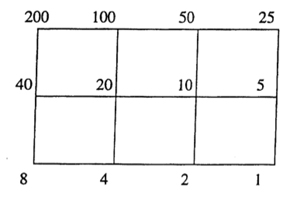As $\Gamma_0(N)$ fixes $L_1$ and $L_N$ it also fixes all lattices in the (N|1)-thread, that is all lattices occurring in a shortest path from $L_1$ to $L_N$ (on the left a picture of the (200|1)-thread).

If $N=p_1^{a_1} p_2^{a_2} \ldots p_k^{a_k}$, then the (N|1)-thread has $2^k$ involutions as symmetries, called the Atkin-Lehner involutions. For every exact divisor $e || N$ (that is, $e|N$ and $gcd(e,\frac{N}{e})=1$ we have an involution $W_e$ which acts by sending each point in the thread-cell corresponding to the prime divisors of $e$ to its antipodal cell-point and acts as the identity on the other prime-axes. For example, in the (200|1)-thread on the left, $W_8$ is the left-right reflexion, $W_{25}$ the top-bottom reflexion and $W_{200}$ the antipodal reflexion. The set of all exact divisors of N becomes the group $~(\mathbb{Z}/2\mathbb{Z})^k$ under the operation $e \ast f = \frac{e \times f}{gcd(e,f)^2}$.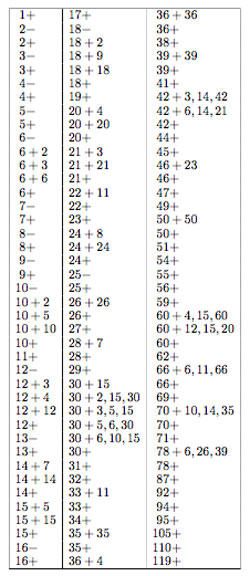Most of the moonshine groups are of the form $\Gamma_0(n|h)+e,f,g,…$ for some $N=h.n$ such that $h | 24$ and $h^2 | N$. The group $\Gamma_0(n|h)$ is then conjugate to the modular subgroup $\Gamma_0(\frac{n}{h})$ by the element $\begin{bmatrix} h & 0 \ 0 & 1 \end{bmatrix}$. With $\Gamma_0(n|h)+e,f,g,…$ we mean that the group $\Gamma_0(n|h)$ is extended with the involutions $W_e,W_f,W_g,…$. If we simply add all Atkin-Lehner involutions we write $\Gamma_0(n|h)+$ for the resulting group.

Finally, whenever $h \not= 1$ there is a subgroup $\Gamma_0(n||h)+e,f,g,…$ which is the kernel of a character $\lambda$ being trivial on $\Gamma_0(N)$ and on all involutions $W_e$ for which every prime dividing $e$ also divides $\frac{n}{h}$, evaluating to $e^{\frac{2\pi i}{h}}$ on all cosets containing $\begin{bmatrix} 1 & \frac{1}{h} \ 0 & 1 \end{bmatrix}$ and to $e^{\pm \frac{2 \pi i }{h}}$ for cosets containing $\begin{bmatrix} 1 & 0 \ n & 0 \end{bmatrix}$ (with a + sign if $\begin{bmatrix} 0 & -1 \ N & 0 \end{bmatrix}$ is present and a – sign otherwise). Btw. it is not evident at all that this is a character, but hard work shows it is!

Clearly there are heavy restrictions on the numbers that actually occur in moonshine. In the paper On the discrete groups of moonshine, John Conway, John McKay and Abdellah Sebbar characterized the 171 arithmetic subgroups of $PSL_2(\mathbb{R})$ occuring in monstrous moonshine as those of the form $G = \Gamma_0(n || h)+e,f,g,…$ which are

• (a) of genus zero, meaning that the quotient of the upper-half plane by the action of $G \subset PSL_2(\mathbb{R})$ by Moebius-transformations gives a Riemann surface of genus zero,
• (b) the quotient group $G/\Gamma_0(nh)$ is a group of exponent 2 (generated by some Atkin-Lehner involutions), and
• (c) every cusp can be mapped to $\infty$ by an element of $PSL_2(\mathbb{R})$ which conjugates the group to one containing $\Gamma_0(nh)$.

Now, if $\Gamma_0(n || h)+e,f,g,…$ is of genus zero, so is the larger group $\Gamma_0(n | h)+e,f,g,…$, which in turn, is conjugated to the group $\Gamma_0(\frac{n}{h})+e,f,g,…$. Therefore, we need a list of all groups of the form $\Gamma_0(\frac{n}{h})+e,f,g,…$ which are of genus zero. There are exactly 123 of them, listed on the right.

How does this help to determine the structure of the p-subtree of the moonshine picture for the fifteen monster-primes p? Look for the largest p-power $p^k$ such that $p^k+e,f,g…$ appears in the list. That is for p=2,3,5,7,11,13,17,19,23,29,31,41,47,59,71 these powers are resp. 5,3,2,2,1,1,1,1,1,1,1,1,1,1,1. Next, look for the largest p-power $p^l$ dividing 24 (that is, 3 for p=2, 1 for p=3 and 0 for all other primes). Then, these relevant moonshine groups contain the modular subgroup $\Gamma_0(p^{k+2l})$ and are contained in its normalizer in $PSL_2(\mathbb{R})$ which by the Atkin-Lehner theorem is precisely the group $\Gamma_0(p^{k+l}|p^l)+$.

Right, now the lattices fixed by $\Gamma_0(p^{k+2l})$ (and permuted by its normalizer), that is the lattices in our p-subtree, are those that form the $~(p^{k+2l}|1)$-snake in Conway-speak. That is, the lattices whose hyper-distance to the $~(p^{k+l}|p^l)$-thread divides 24. So for all primes larger than 2 or 3, the p-tree is just the $~(p^l|1)$-thread.

For p=3 the 3-tree is the (243|1)-snake having the (81|3)-thread as its spine. It contains the following lattices, all of which are number-like.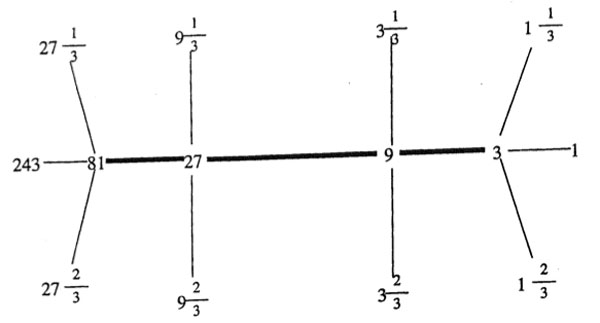Depicting the 2-tree, which is the (2048|1)-snake may take a bit longer… Perhaps someone should spend some time figuring out which cells of the product of these fifteen trees make up the moonshine picture!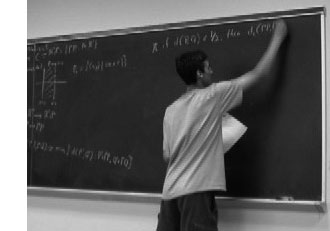Charles Siegel of Rigorous Trivialities ran a great series on big theorems.

The series started january 10th 2008 with a post on Bezout’s theorem, followed by posts on Chow’s lemma, Serre duality, Riemann-Roch, Bertini, Nakayama’s lemma, Groebner bases, Hurwitz to end just before christmas with a post on Kontsevich’s formula.

Also at other blogs, 2008 was the year of series of long posts containing substantial pure mathematics.

Out of many, just two examples : Chris Schommer-Pries ran a three part series on TQFTs via planar algebras starting here, at the secret blogging seminar.
And, Peter Woit of Not Even Wrong has an ungoing series of posts called Notes on BRST, starting here. At the moment he is at episode nine.

It suffices to have a quick look at the length of any of these posts, to see that a great deal of work was put into these series (and numerous similar ones, elsewhere). Is this amount of time well spend? Or, should we focus on shorter, easier digestible math-posts?

What got me thinking was this merciless comment Charles got after a great series of posts leading up to Kontsevich’s formula :

“Perhaps you should make a New Years commitment to not be so obscurantist, like John Armstrong, and instead promote the public understanding of math!”

Well, if this doesn’t put you off blogging for a while, what will?

So, are we really writing the wrong sort of posts? Do math-blog readers only want short, flashy, easy reading posts these days? Or, is anyone out there taking notice of the hard work it takes to write such a technical post, let alone a series of them?

At first I was rather pessimistic about the probable answer to all these questions, but, fortunately we have Google Analytics to quantify things a bit.

Clearly I can only rely on the statistics for my own site, so I’ll treat the case of a recent post here : Mumford’s treasure map which tried to explain the notion of a generic point and how one might depict an affine scheme.

Here’s some of the Google Analytics data :The yellow function gives the number of pageviews for that post, the value ranges between 0 and 600 (the number to the right of the picture). In total this post was viewed 2470 times, up till now.

The blue function tells the average time a visitor spend reading that post, the numbers range between 0 and 8 minutes (the times to the left of the picture). On average the time-on-page was 2.24 minutes, so in all people spend well over 92 hours reading this one post! This seems like a good return for the time it took me to write it…

Some other things can be learned from this data. Whereas the number of page-views has two peaks early on (one the day it was posted, the second one when Peter Woit linked to it) and is now steadily decreasing, the time-on-page for the later visitors is substantially longer than the early readers.

Some of this may be explained (see comment below) by returning visits. Here is a more detailed picture (orange = new visits, green=returning visits, blue=’total’ whatever this means).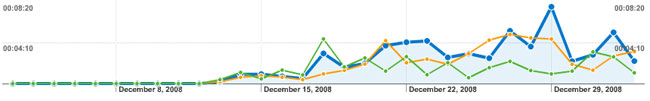All in all good news : there is indeed a market for longer technical math-posts and people (eventually) take time to read the post in detail.